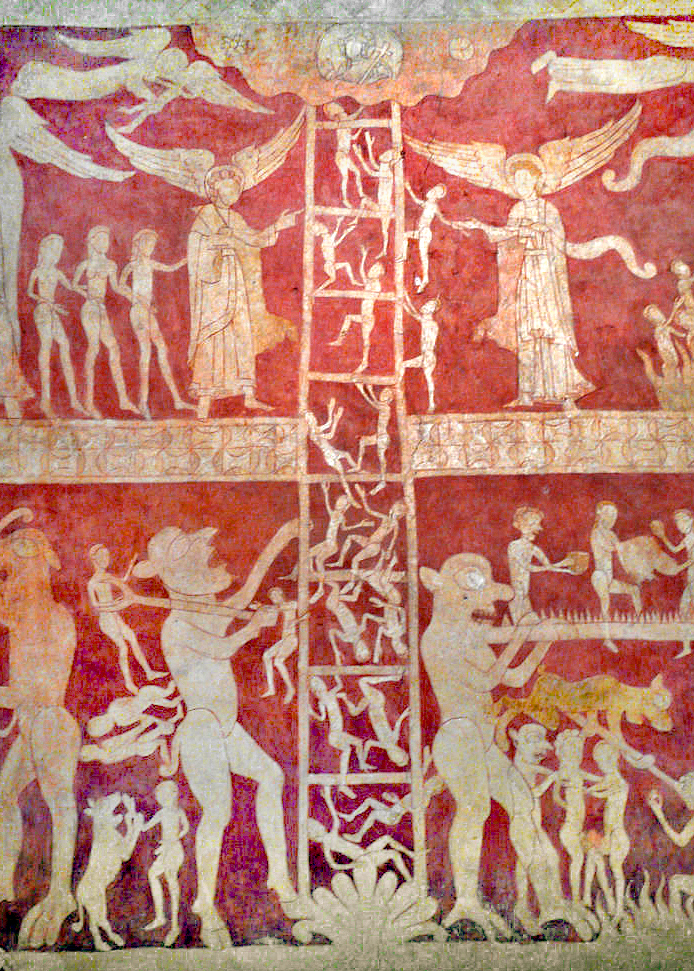# Pointwise definable and Leibnizian models of arithmetic and set theory, realized in end extensions of a given model, Notre Dame Logic Seminar, October 2022

This will be a talk for the Notre Dame logic seminar, 11 October 2022, 2pm in Hales-Healey Hall.

Abstract.  I shall present very new results on pointwise definable and Leibnizian end-extensions of models of arithmetic and set theory. Using the universal algorithm, I shall present a new flexible method showing that every countable model of PA admits a pointwise definable $\Sigma_n$-elementary end-extension. Also, any model of PA of size at most continuum admits an extension that is Leibnizian, meaning that any two distinct points are separated by some expressible property. Similar results hold in set theory, where one can also achieve V=L in the extension, or indeed any suitable theory holding in an inner model of the original model.

# An infinitary-logic-free proof of the Barwise end-extension theorem, with new applications, University of Münster, January 2019

This will be a talk for the Logic Oberseminar at the University of Münster, January 11, 2019.Abstract. I shall present a new proof, with new applications, of the amazing extension theorem of Barwise (1971), which shows that every countable model of ZF has an end-extension to a model of ZFC + V=L. This theorem is both (i) a technical culmination of Barwise’s pioneering methods in admissible set theory and the admissible cover, but also (ii) one of those rare mathematical results saturated with significance for the philosophy of set theory. The new proof uses only classical methods of descriptive set theory, and makes no mention of infinitary logic. The results are directly connected with recent advances on the universal $\Sigma_1$-definable finite set, a set-theoretic version of Woodin’s universal algorithm.# A new proof of the Barwise extension theorem, without infinitary logic, CUNY Logic Workshop, December 2018

I’ll be back in New York from Oxford, and this will be a talk for the CUNY Logic Workshop, December 14, 2018.Abstract. I shall present a new proof, with new applications, of the amazing extension theorem of Barwise (1971), which shows that every countable model of ZF has an end-extension to a model of ZFC + V=L. This theorem is both (i) a technical culmination of Barwise’s pioneering methods in admissible set theory and the admissible cover, but also (ii) one of those rare mathematical results saturated with significance for the philosophy of set theory. The new proof uses only classical methods of descriptive set theory, and makes no mention of infinitary logic. The results are directly connected with recent advances on the universal $\Sigma_1$-definable finite set, a set-theoretic version of Woodin’s universal algorithm.

# Parallels in universality between the universal algorithm and the universal finite set, Oxford Math Logic Seminar, October 2018

This will be a talk for the Logic Seminar in Oxford at the Mathematics Institute in the Andrew Wiles Building on October 9, 2018, at 4:00 pm, with tea at 3:30.Abstract. The universal algorithm is a Turing machine program $e$ that can in principle enumerate any finite sequence of numbers, if run in the right model of PA, and furthermore, can always enumerate any desired extension of that sequence in a suitable end-extension of that model. The universal finite set is a set-theoretic analogue, a locally verifiable definition that can in principle define any finite set, in the right model of set theory, and can always define any desired finite extension of that set in a suitable top-extension of that model. Recent work has uncovered a $\Sigma_1$-definable version that works with respect to end-extensions. I shall give an account of all three results, which have a parallel form, and describe applications to the model theory of arithmetic and set theory.

Slides